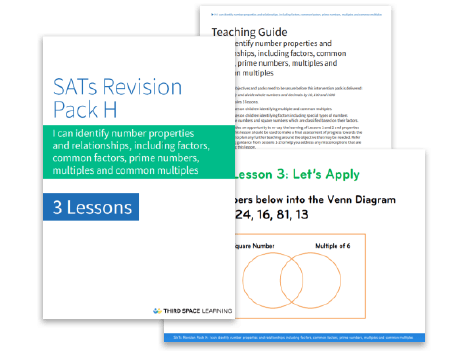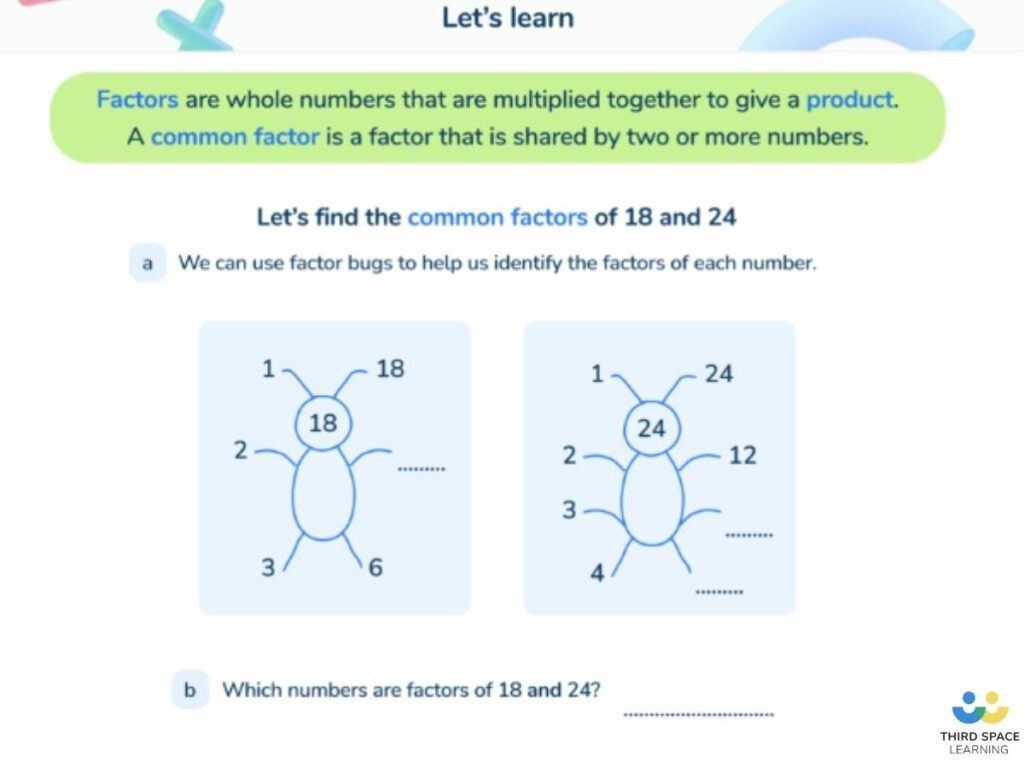Teaching support from the UK’s largest provider of in-school maths tuitionone to one lessonsschools supported

Consolidate learning and plug gaps

In-school online one to one maths tuition developed by maths teachers and pedagogy experts

See it in action

Hundreds of FREE online maths resources!

Daily activities, ready-to-go lesson slides, SATs revision packs, video CPD and more!Explore all resources
Four Operations

Consolidate learning and plug gaps

In-school online one to one maths tuition developed by maths teachers and pedagogy experts

See it in action

# What Is The Highest Common Factor: Explained For Primary Parents And Kids

In this post, we will be answering the question “what is the highest common factor” and providing you with all of the information you need to help students understand this area of maths. We’ve also got some questions based around the Highest Common Factor that students can complete.FREE Factors, Multiples, Square & Cube Numbers Pack

Download this resource pack aimed at helping pupils identify number properties and relationships in advance of SATs. It includes teaching guidance, pupil practice sheets and activity slides.Download Free Now!

### What is a factor in maths?

Factors are numbers that fits exactly into other number. For example, 5 is a factor of 10; 7 is a factor of 28. One way of helping children remember factors is to think of factories – factories make things, and factors make up numbers.

Another way is to think of them in pairs (factors are friends – they come in pairs!); for example, 5 x 2 = 10, so 5 and 2 are factors of 10; 7 x 4 = 28, so 7 and 4 are factors of 28. There are 8 factors of 24: 1, 2, 3, 4, 6, 8, 12 and 24 (1 x 24, 2 x 12, 3 x 8 and 4 x 6). Factors are whole numbers rather than decimals.

Children often confuse factors with multiples, so it is important they learn about the difference between factors and multiples.

### What is a common factor?

A common factor is a factor that is shared by multiple numbers. 7 is a common factor of 14 and 21, as it fits exactly into both. Another example would be the common factors of 8 and 12 which are 1, 2 and 4.

A visual way to see common factors is by using factor trees for each of the numbers you are trying to find common factors for. This will allow you to see both the larger numbers and smaller numbers which are common. Another method is writing out each of the factors for the given numbers and underlining any factors which are common.A slide teaching pupils how to find common factors between numbers from Third Space Learning’s online intervention.

### What is the highest common factor?

The highest common factor is the largest whole number which is shared by given numbers. For example, common factors of 10 and 20 are 1, 2, 5 and 10, but the highest of those is 10; therefore, the highest common factor of 10 and 20 is 10. Other terms can sometimes be used, mainly greatest common factor (GCF) and greatest common divisor (GCD).

### How to find the highest common factor

One quick and easy way of helping children to find the highest common factor is to ask them to list the factors of each number, underline all the common factors and then find the largest number. For example, the HCF of 18 and 24 is 6:

Another example is that the highest common factor of 21, 36 and 42 is 3:

### When will my child learn about the highest common factors?

Factors are introduced in Year 4. The national curriculum states that Year 4 pupils should be taught to recognise and use factor pairs. The non-statutory guidance suggests that pupils use factors and multiples to recognise equivalent fractions and simplify where appropriate (for example, 6/9 = 2/3 or 1/4 = 2/8).

This is then extended to common factors in Year 5. The national curriculum states that Year 5 pupils should be taught to identify factors, including finding all factor pairs of a number, and common factors of two numbers and solve problems involving multiplication and division including using their knowledge of factors.

In Year 6, children are expected to apply their knowledge of common factors to fractions. The national curriculum states that Year 6 pupils should be taught to identify common factors and use common factors to simplify fractions. The non-statutory guidance also suggests that common factors can be related to finding equivalent fractions.

Take a look at our multiplication worksheets that cover all areas of multiplication including long multiplication, factors and multiples.

### How do the highest common factors relate to other areas of maths?

The highest common factors are useful when simplifying and comparing fractions. If you can work out the highest common factor of the numerator (top number) and the denominator (bottom number), you can express the fraction in its simplest form (a skill required in Year 6).

For example, to simplify 18/24, we divide by the numerator and denominator by the HCF of 18 and 24 (6) to get 3/4.

### Highest Common Factor Practice questions

1) Calculate the highest common factor of 24 and 36.

2) Complete this sentence: Every number with a factor of 10 must also have factors of? and ? and ?.

3) Write all the factors of 30 which are also factors of 20.

4) Use your knowledge of the highest common factors to express 48/60 in its simplest form.

Wondering about how to explain other key maths vocabulary to your children? Check out our Primary Maths Dictionary, or try these primary maths terms:

There are plenty of worksheets, tutorials and interactive resources for teaching the highest common multiples (HCF), lowest common multiples (lcm), common prime factors, prime numbers and more on the Third Space Learning Maths Hub.

Do you have pupils who need extra support in maths?
Every week Third Space Learning’s maths specialist tutors support thousands of pupils across hundreds of schools with weekly online 1-to-1 lessons and maths interventions designed to plug gaps and boost progress.

Since 2013 we’ve helped over 150,000 primary and secondary school pupils become more confident, able mathematicians. Learn more or request a personalised quote for your school to speak to us about your school’s needs and how we can help.

Primary school tuition targeted to the needs of each child and closely following the National Curriculum.##### Ellie Williams
Third Space Learning
Content Team
With a love for all things KS2 maths, Ellie is a part of the content team that helps all of the Third Space Learning blogs and resources reach teachers!

Related Articles

x#### FREE Factors, Multiples, Square & Cube Numbers Pack

Download this resource pack aimed at helping pupils identify number properties and relationships in advance of SATs. It includes teaching guidance, pupil practice sheets and activity slides.

#### FREE Factors, Multiples, Square & Cube Numbers PackDownload this resource pack aimed at helping pupils identify number properties and relationships in advance of SATs. It includes teaching guidance, pupil practice sheets and activity slides.